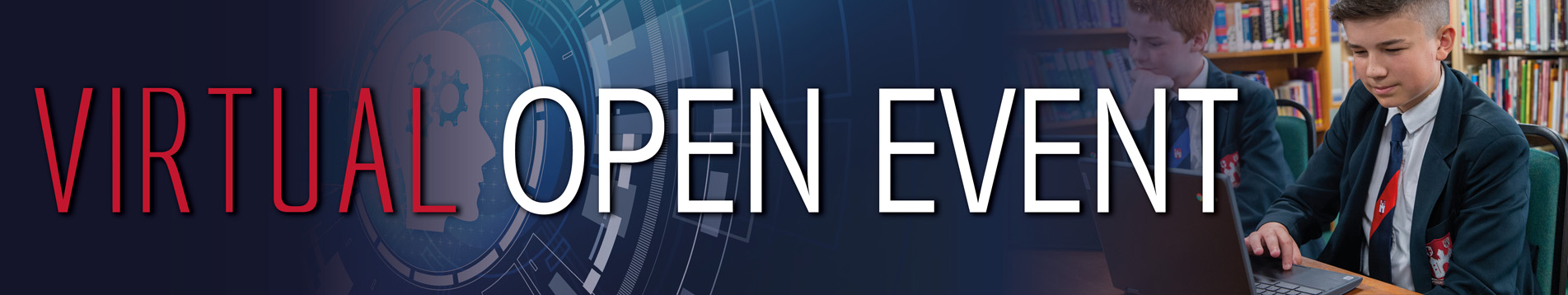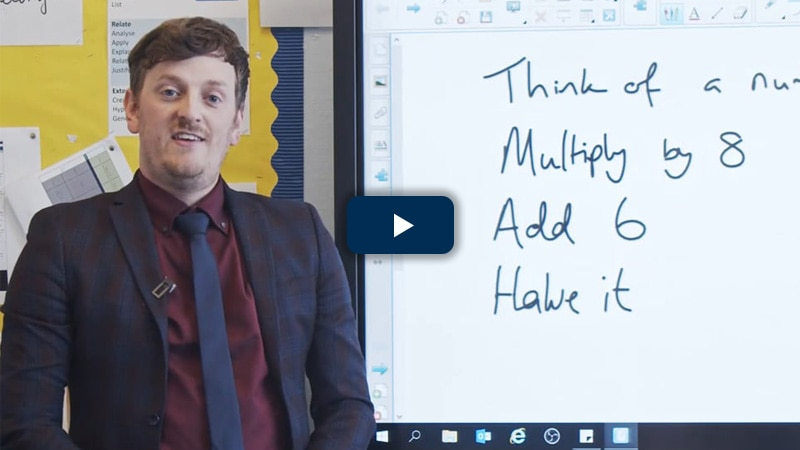At Bridgnorth Endowed, we use the Shanghai Maths Method, an approach that saw the Chinese city topping the table in maths for years and leaving all over countries trailing behind.

This involves teachers using well thought-out mathematical models to develop deep understanding, often involving different ways of thinking about the same concept. Lessons are very interactive, with lots of careful questioning and regular reviews of what’s been learnt.

## Topics include:

• Algebra
• Numbers and the number system
• Counting and comparing
• Multiplication and division
• Geometry and 3D shapes
• Investigating properties of shape
• Exploring fractions, decimals, percentages
• Proportional reasoning
• Pattern sniffing
• Measuring space
• Investigating angles
• Solving equations and inequalities
• Calculating space
• Checking, approximating, estimating
• Mathematical movement
• Presentation and measurement of dataFind out about Maths at BES